• Weather
• Ocean
• Ice & Icebergs
• Other Hazards
• Archive Data
• Digital Forecasts
• Electronic Marine Charting (ECDIS)
• Environmental
• Extratropical Storm Surge Models
• Freezing Spray
• GIS
• Observations
• Ocean
• Probabilistic
• Product Information
• Receiving Products
• About OPC
• News
• Social Media

## GWES Probabilistic Wave Height Guidance

Use the drop down menus below to change the display.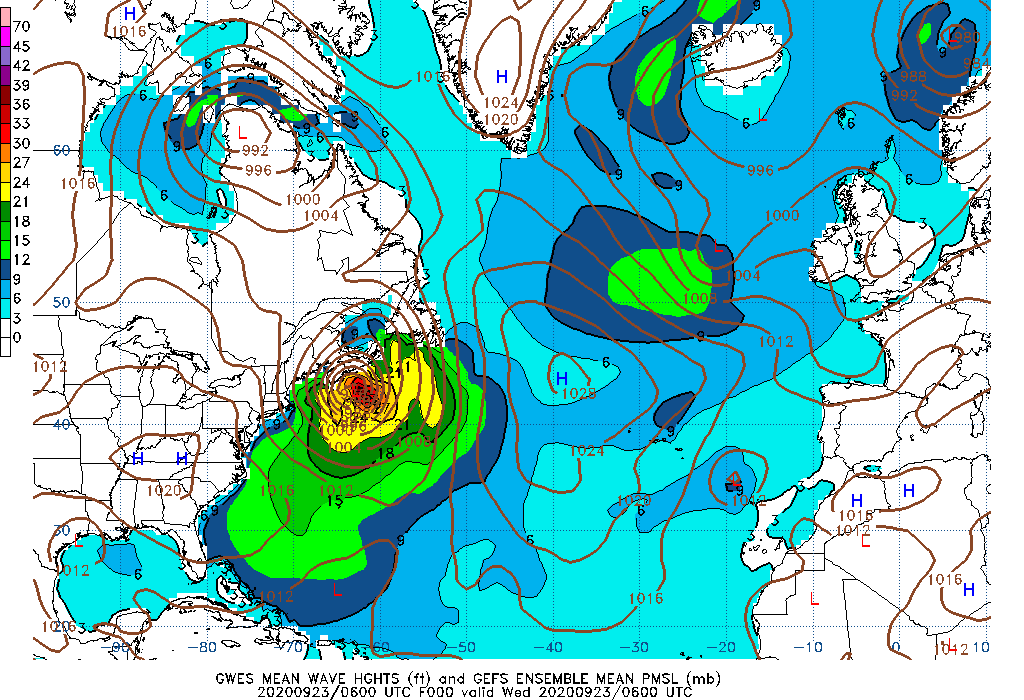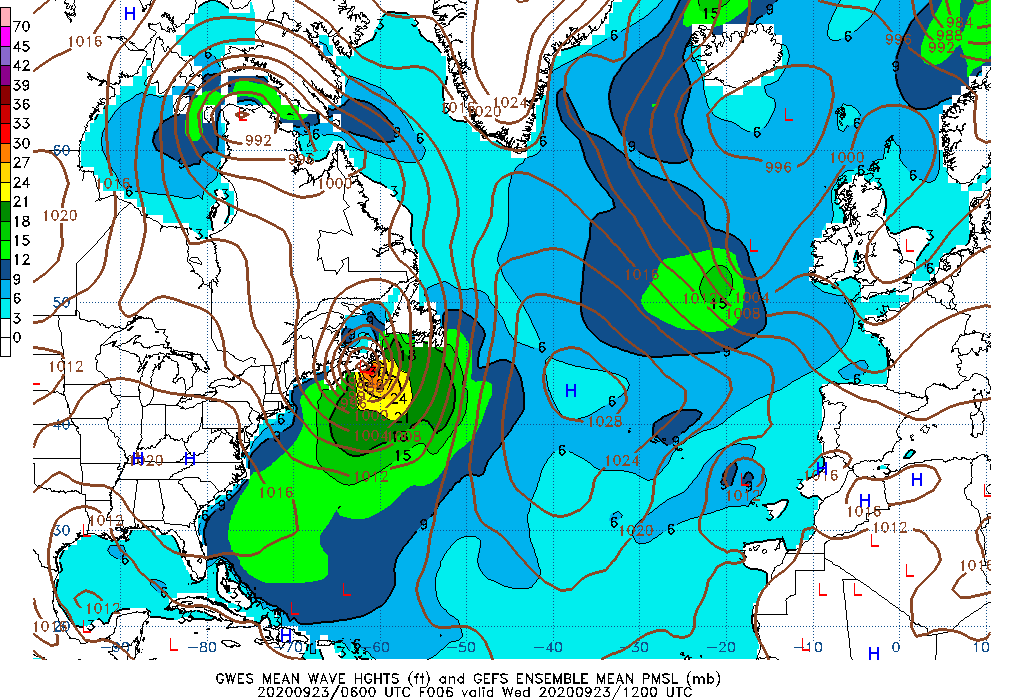000 Hour Wave Height mean 006 Hour Wave Height mean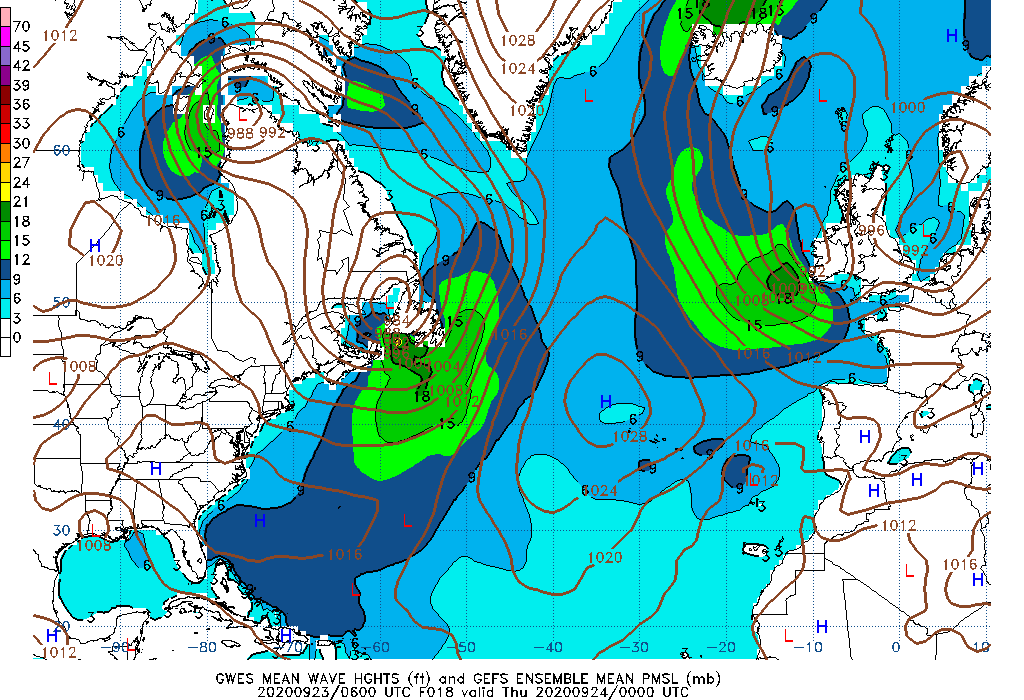012 Hour Wave Height mean 018 Hour Wave Height mean024 Hour Wave Height mean 030 Hour Wave Height mean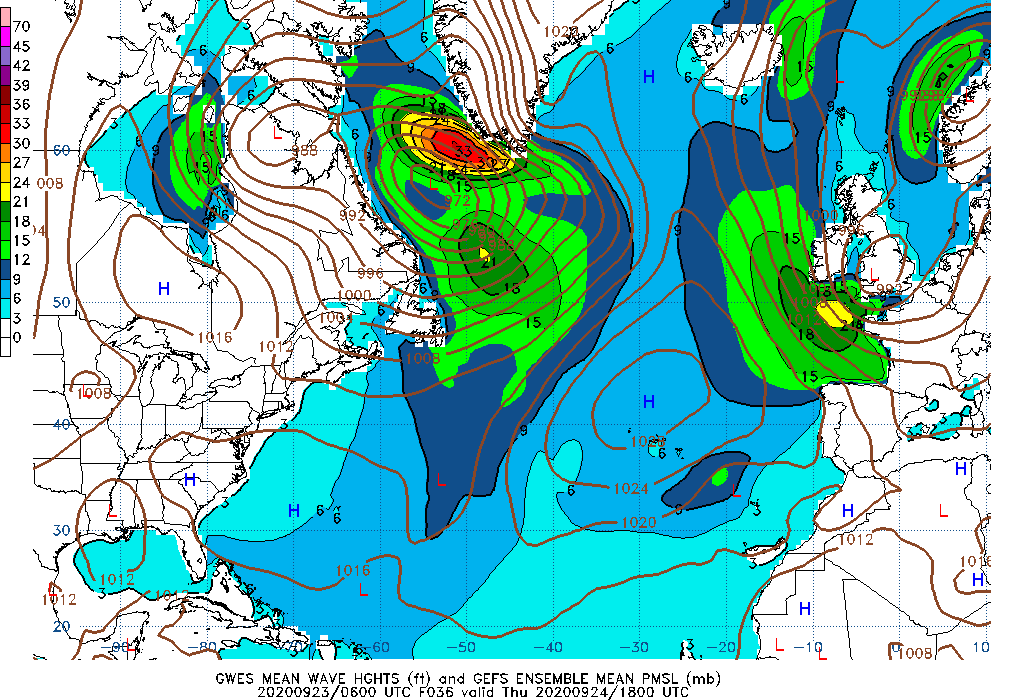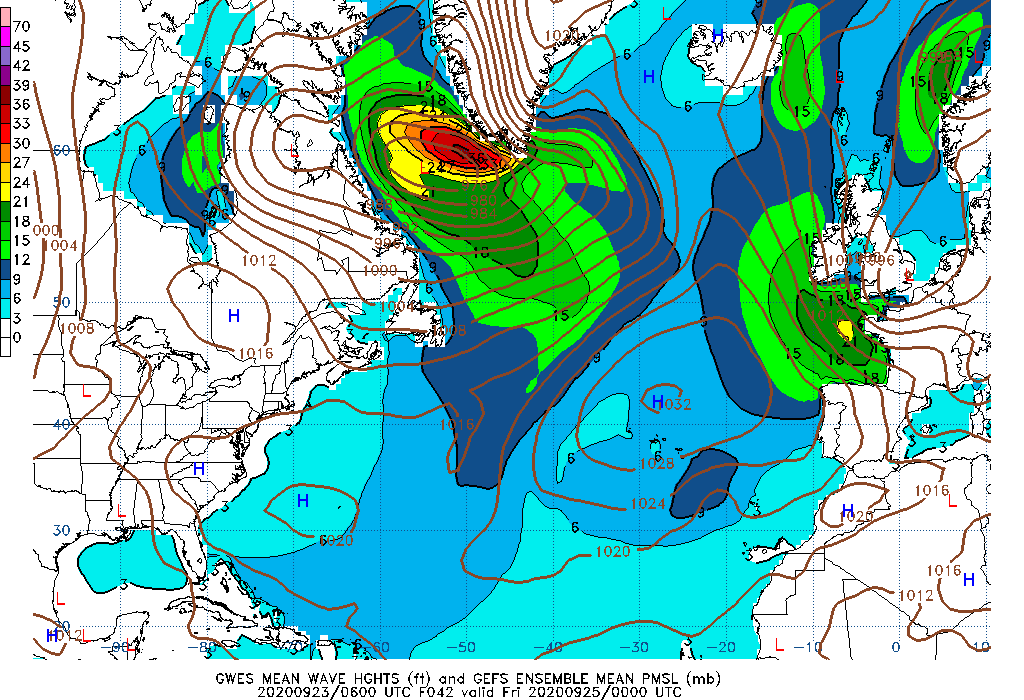036 Hour Wave Height mean 042 Hour Wave Height mean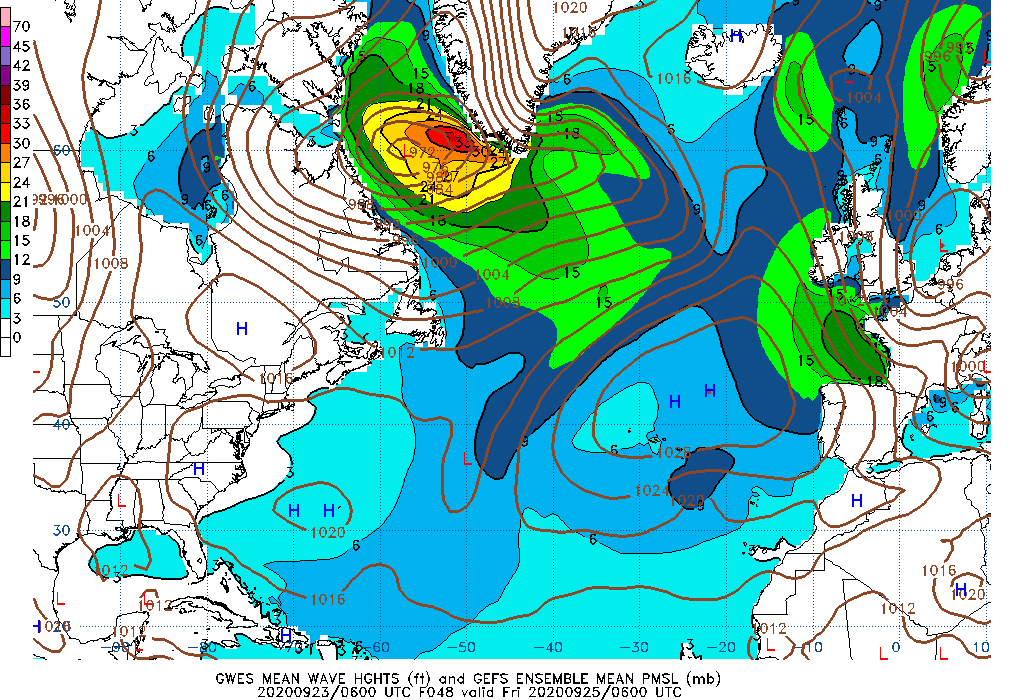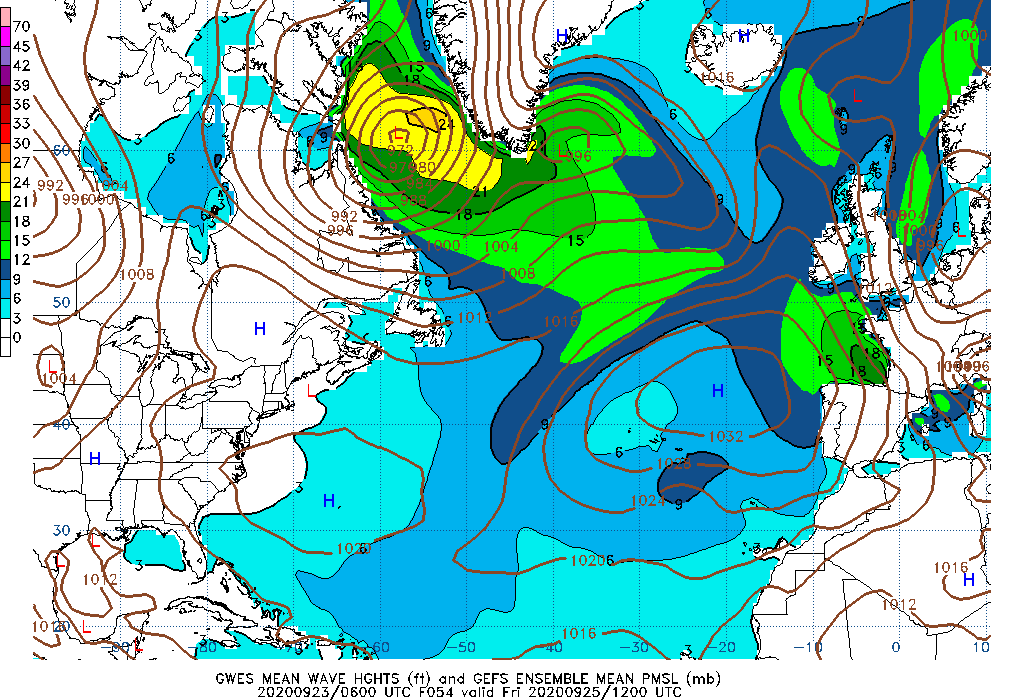048 Hour Wave Height mean 054 Hour Wave Height mean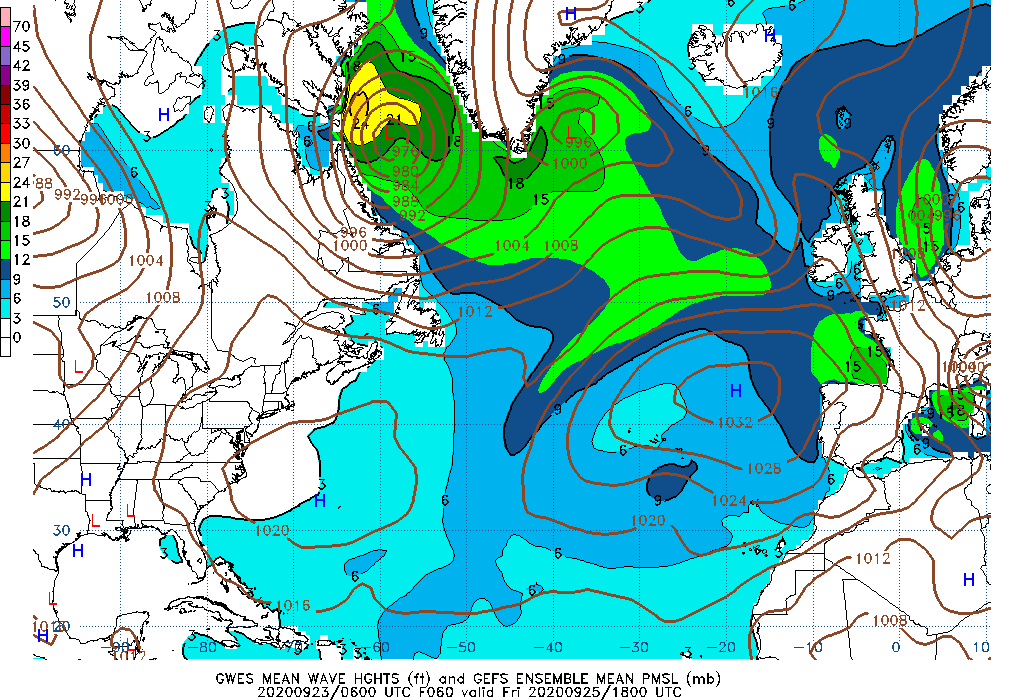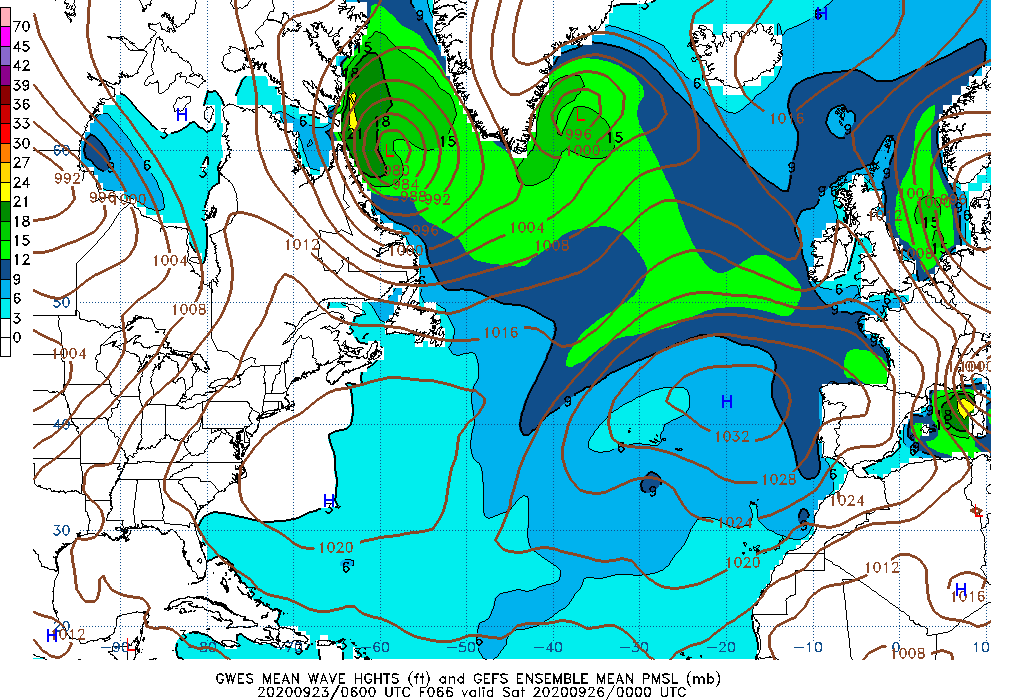060 Hour Wave Height mean 066 Hour Wave Height mean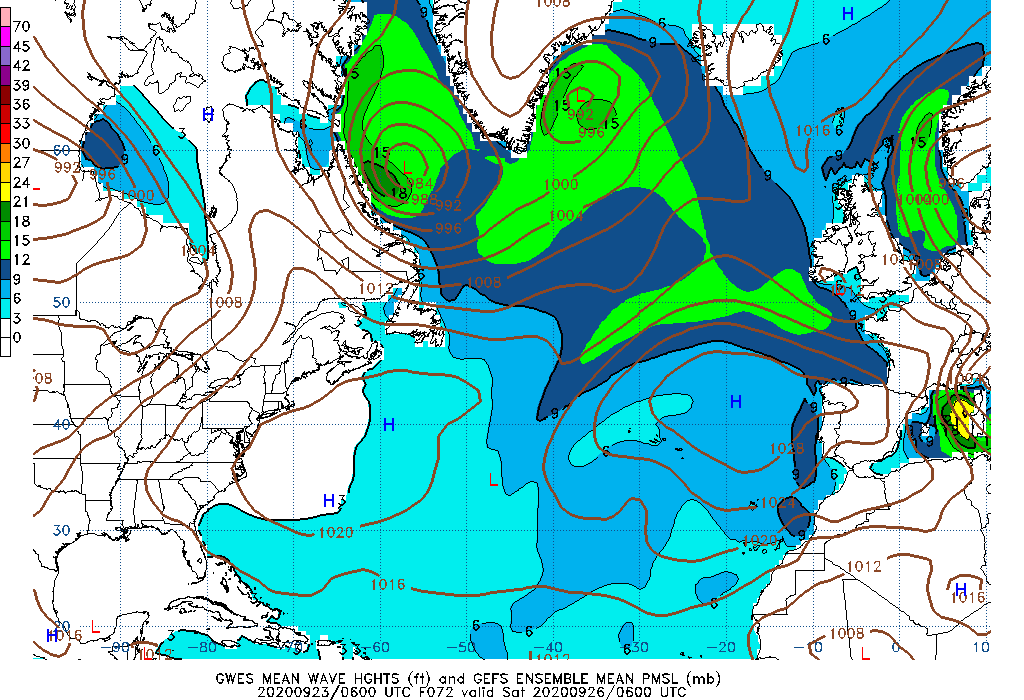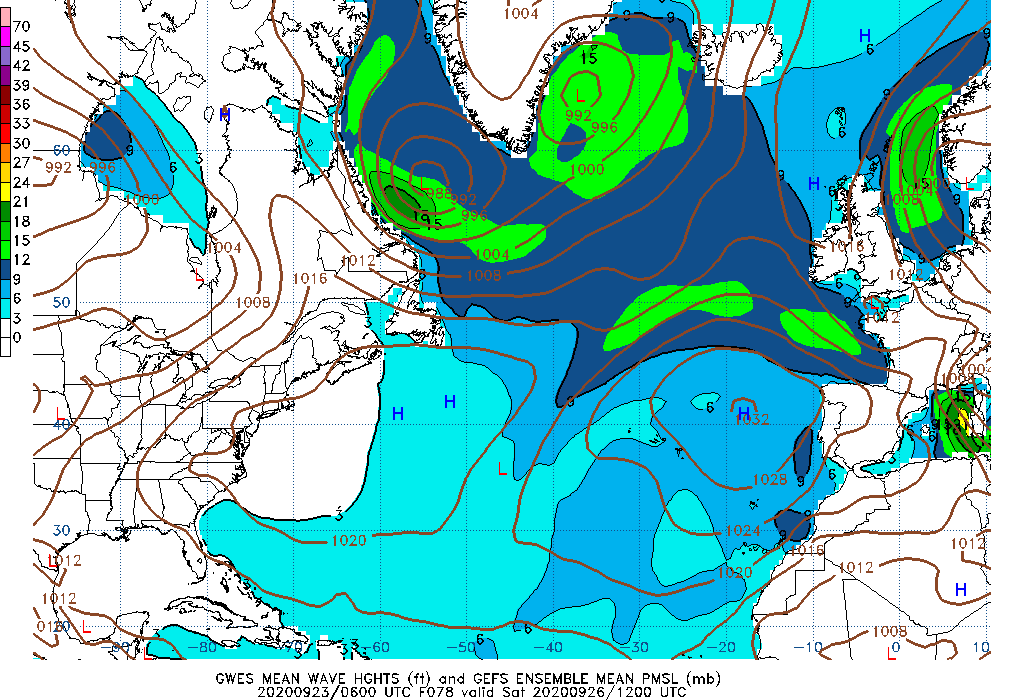072 Hour Wave Height mean 078 Hour Wave Height mean084 Hour Wave Height mean 090 Hour Wave Height mean096 Hour Wave Height mean 102 Hour Wave Height mean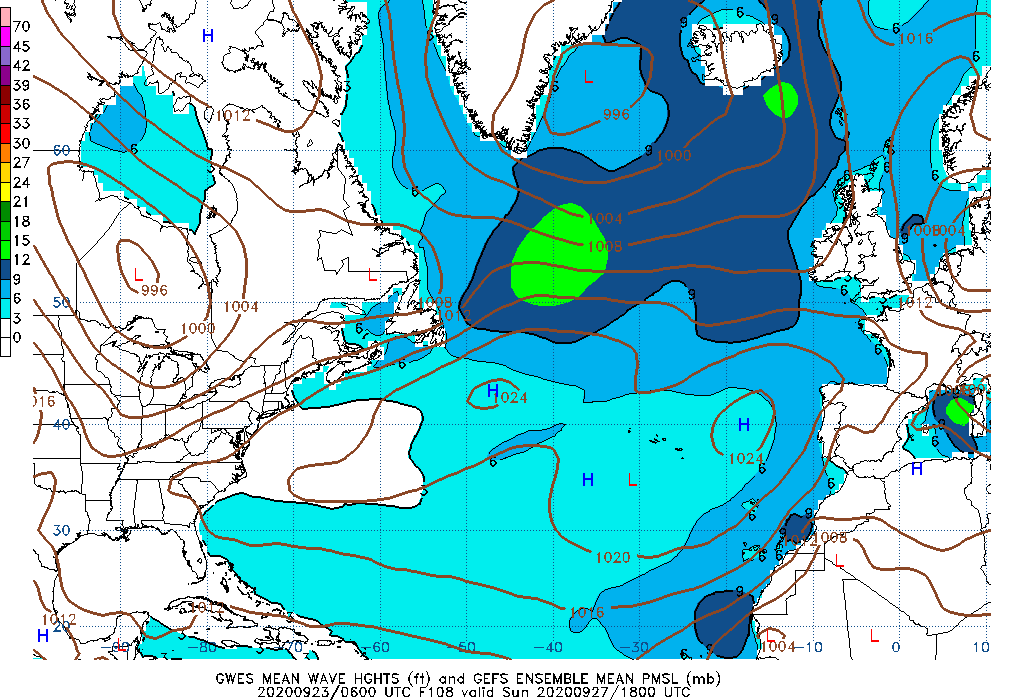108 Hour Wave Height mean 114 Hour Wave Height mean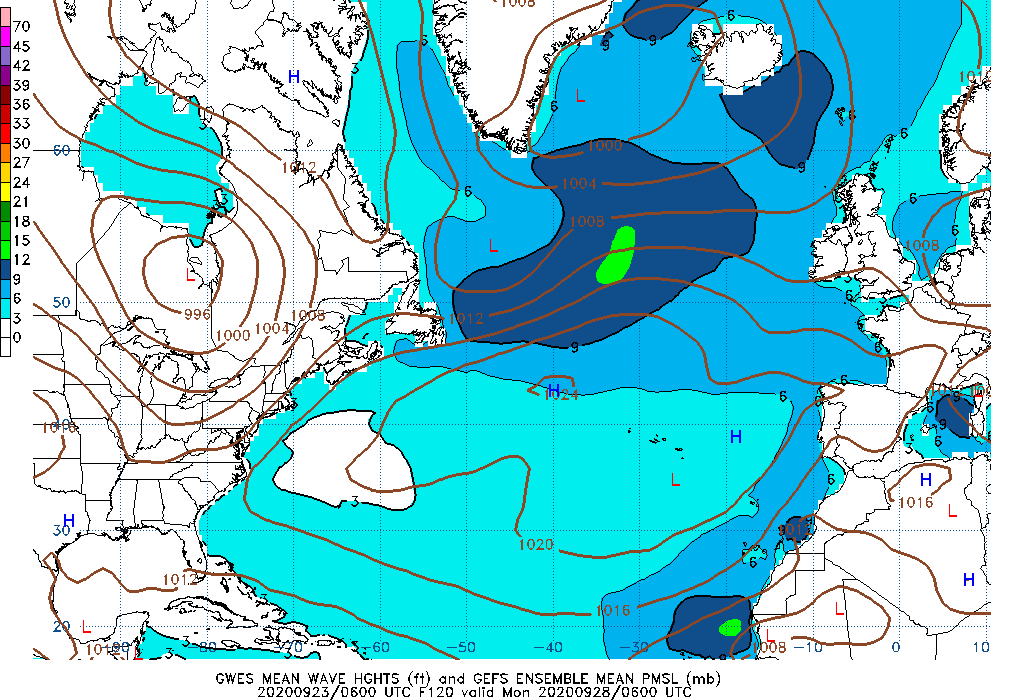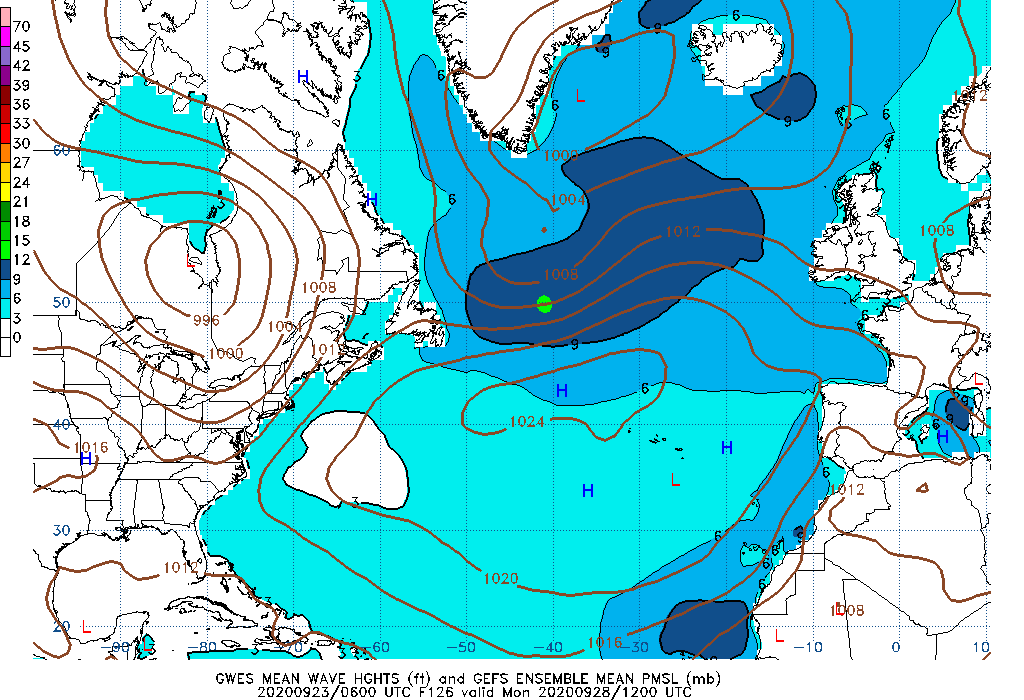120 Hour Wave Height mean 126 Hour Wave Height mean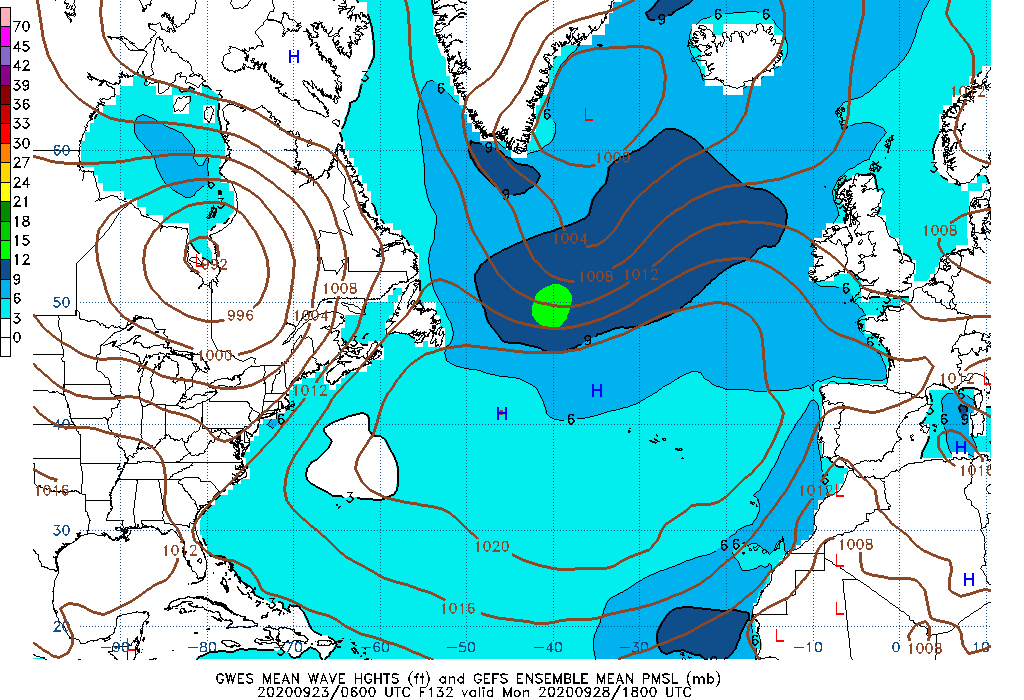132 Hour Wave Height mean 138 Hour Wave Height mean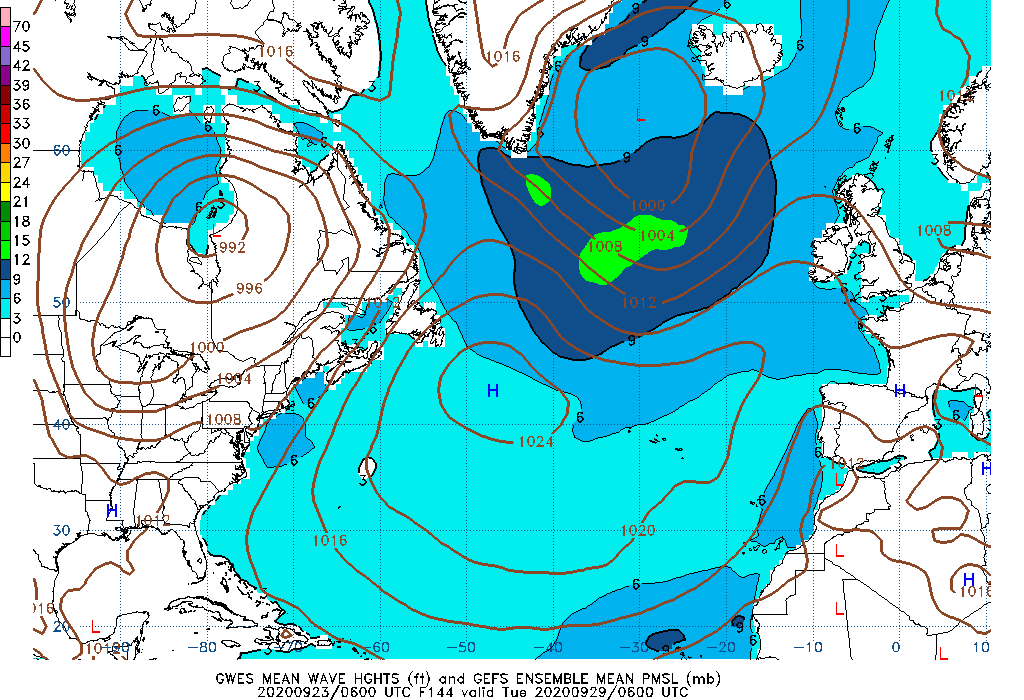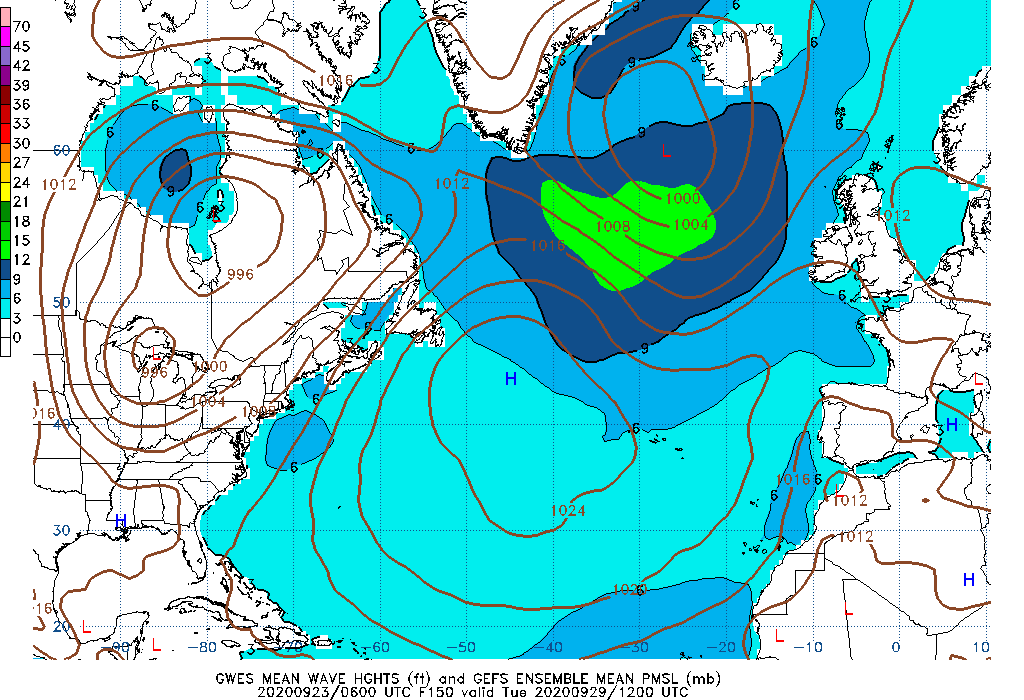144 Hour Wave Height mean 150 Hour Wave Height mean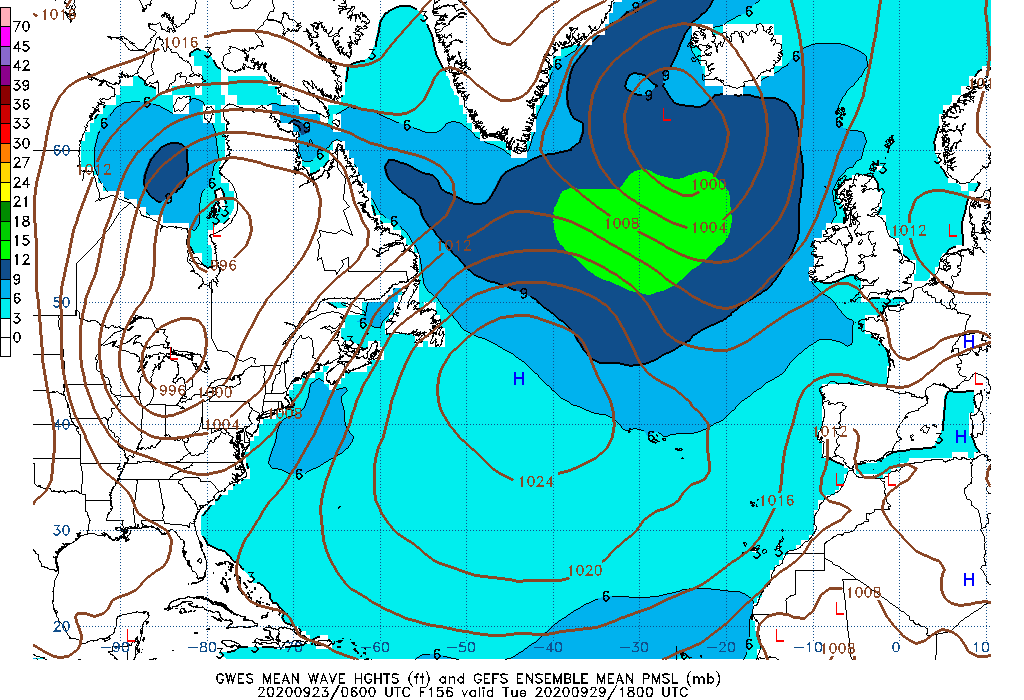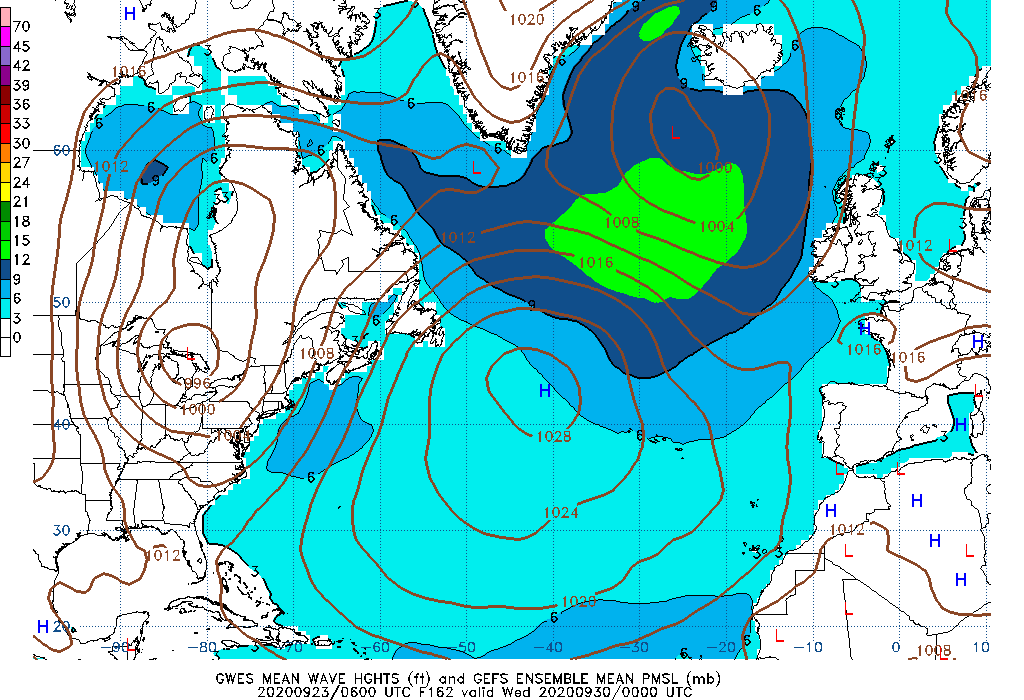156 Hour Wave Height mean 162 Hour Wave Height mean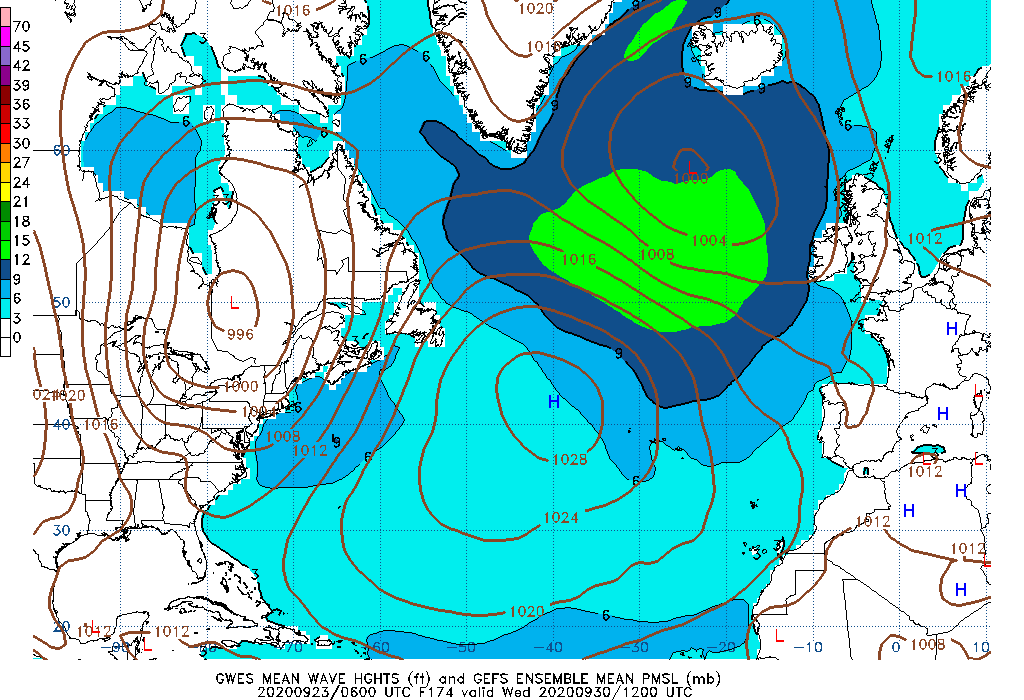168 Hour Wave Height mean 174 Hour Wave Height mean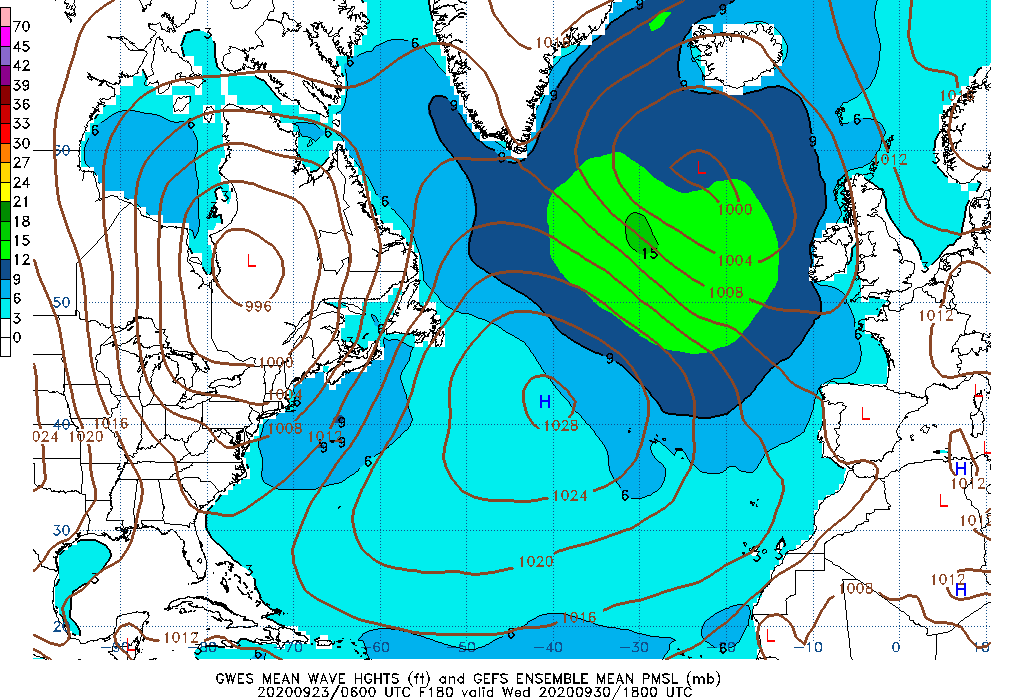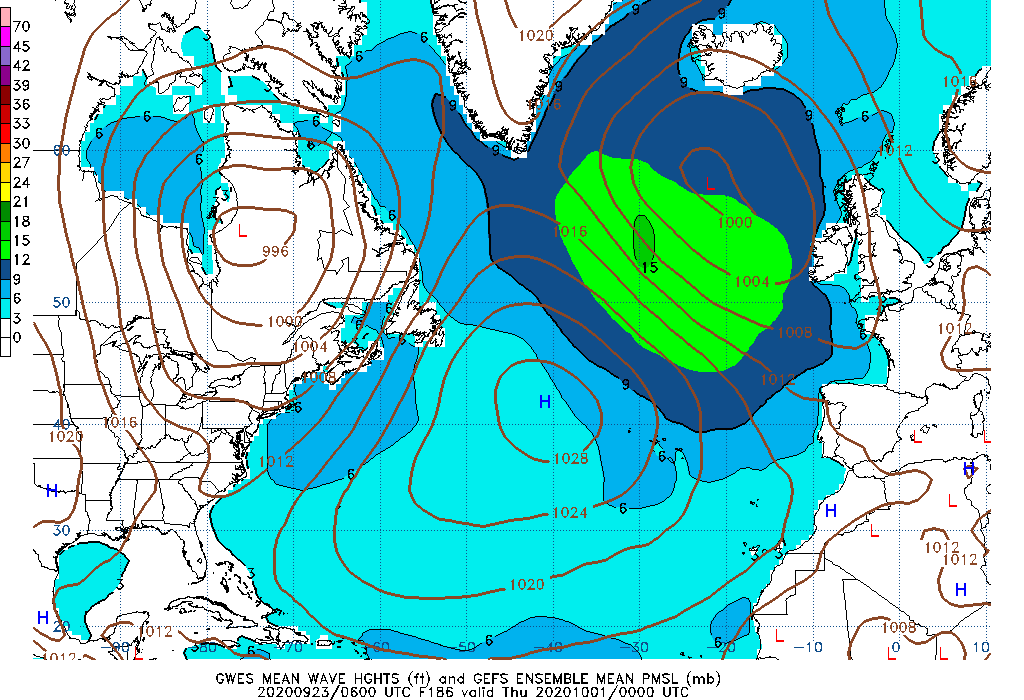180 Hour Wave Height mean 186 Hour Wave Height mean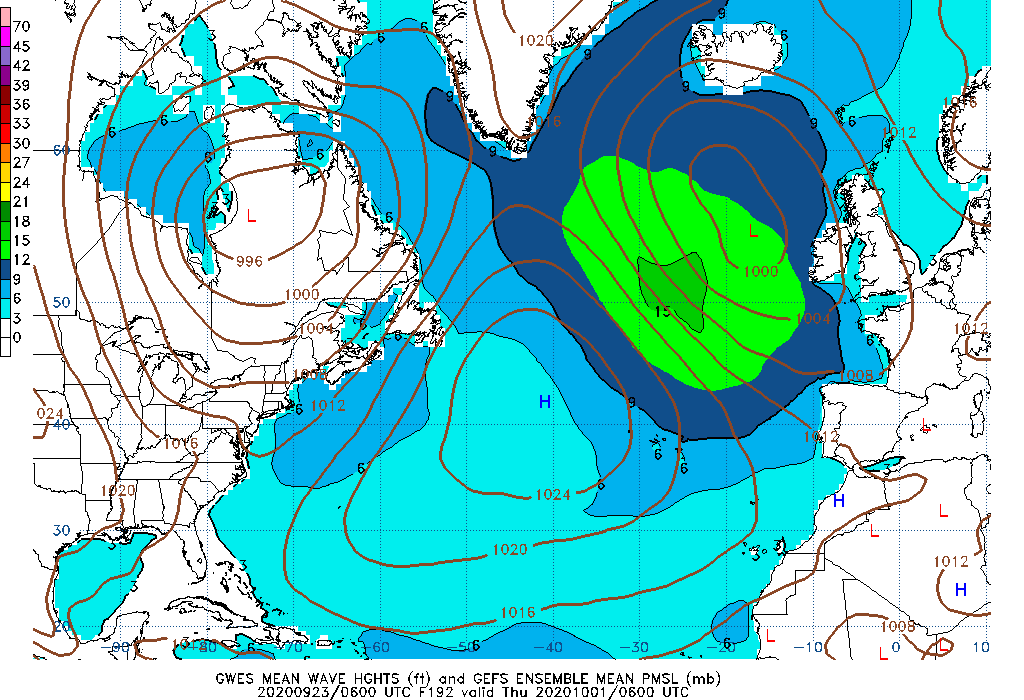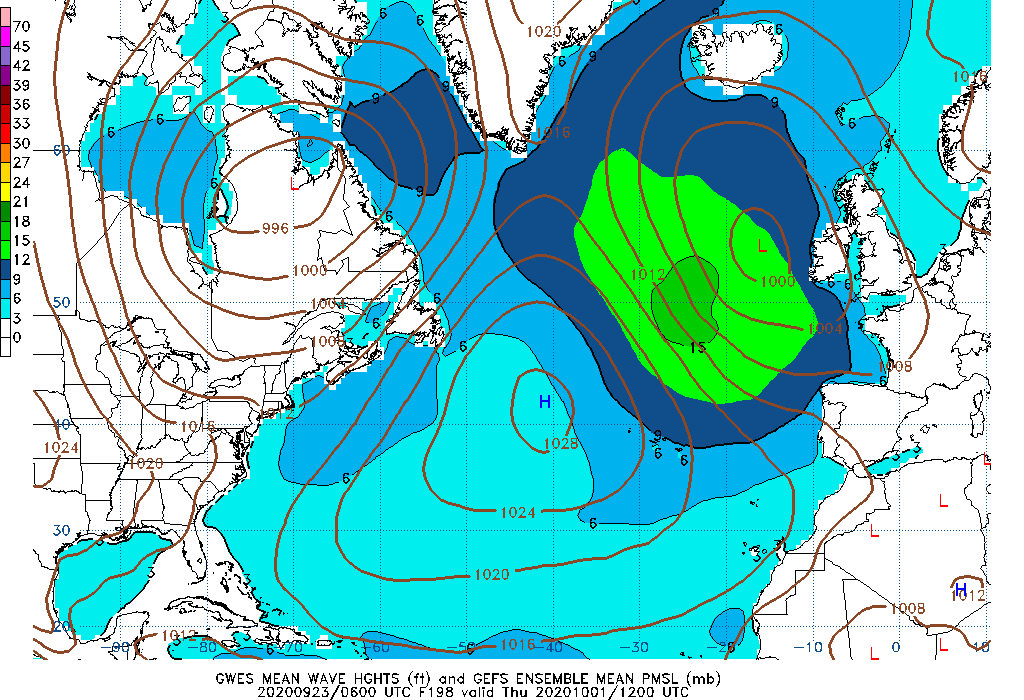192 Hour Wave Height mean 198 Hour Wave Height mean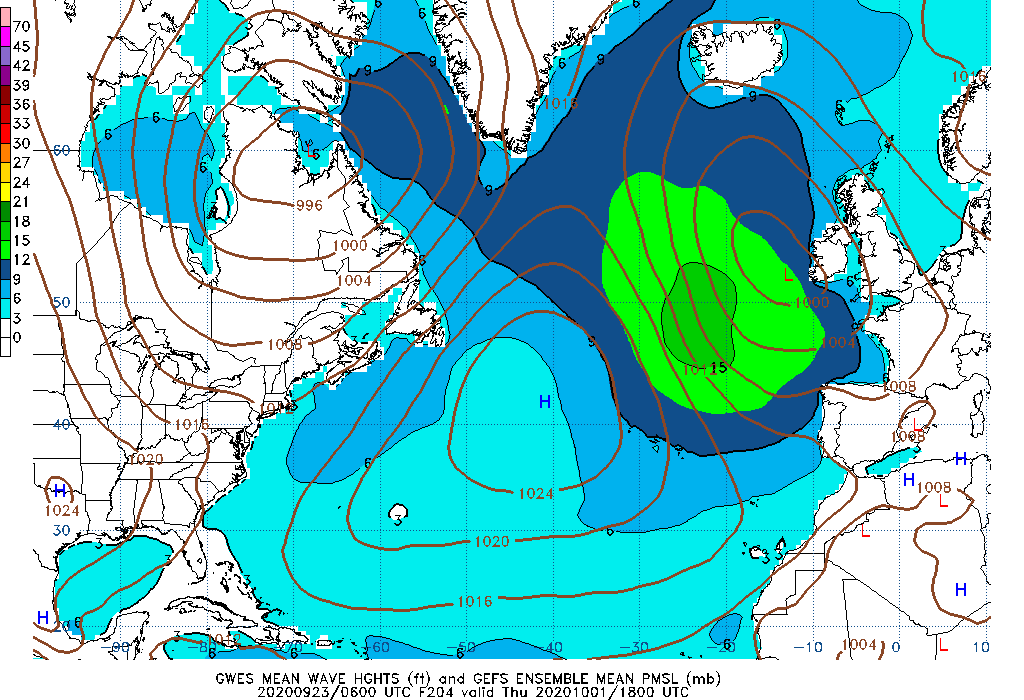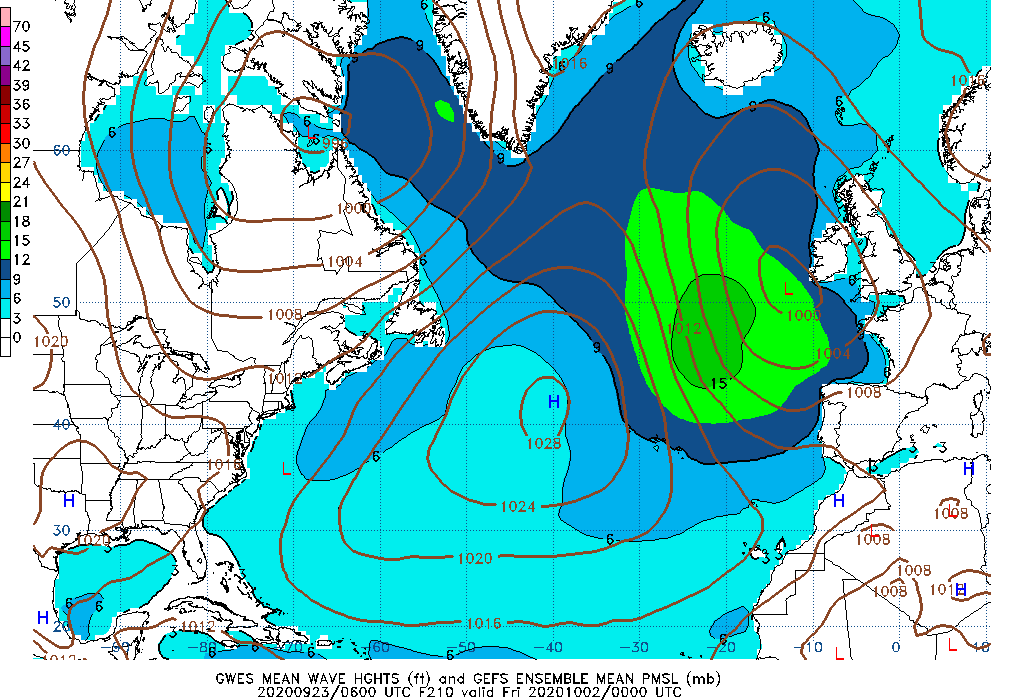204 Hour Wave Height mean 210 Hour Wave Height mean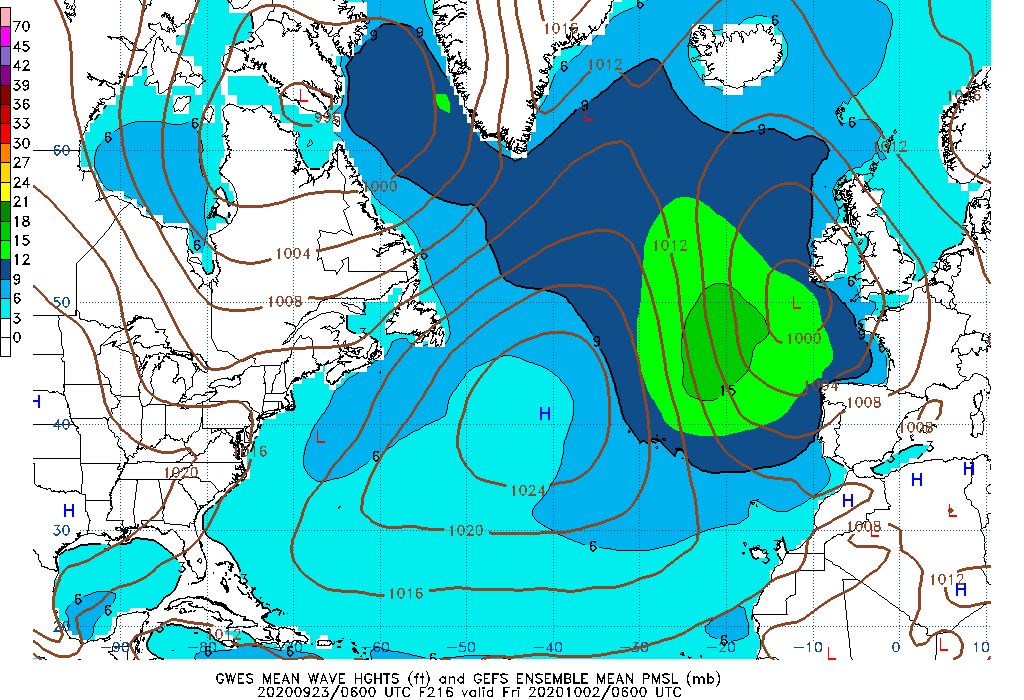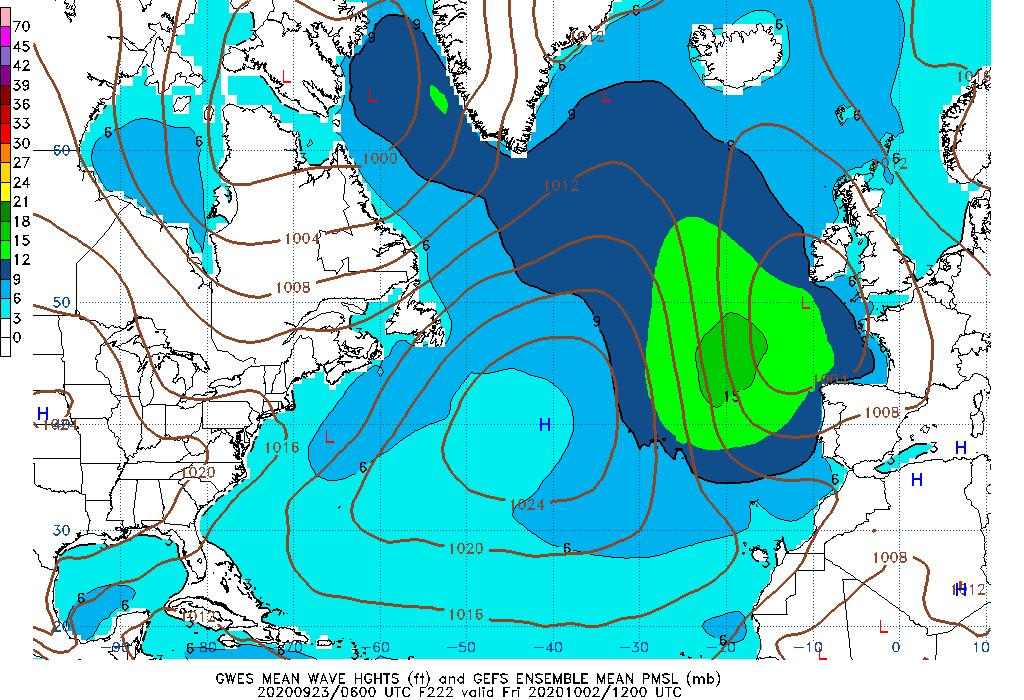216 Hour Wave Height mean 222 Hour Wave Height mean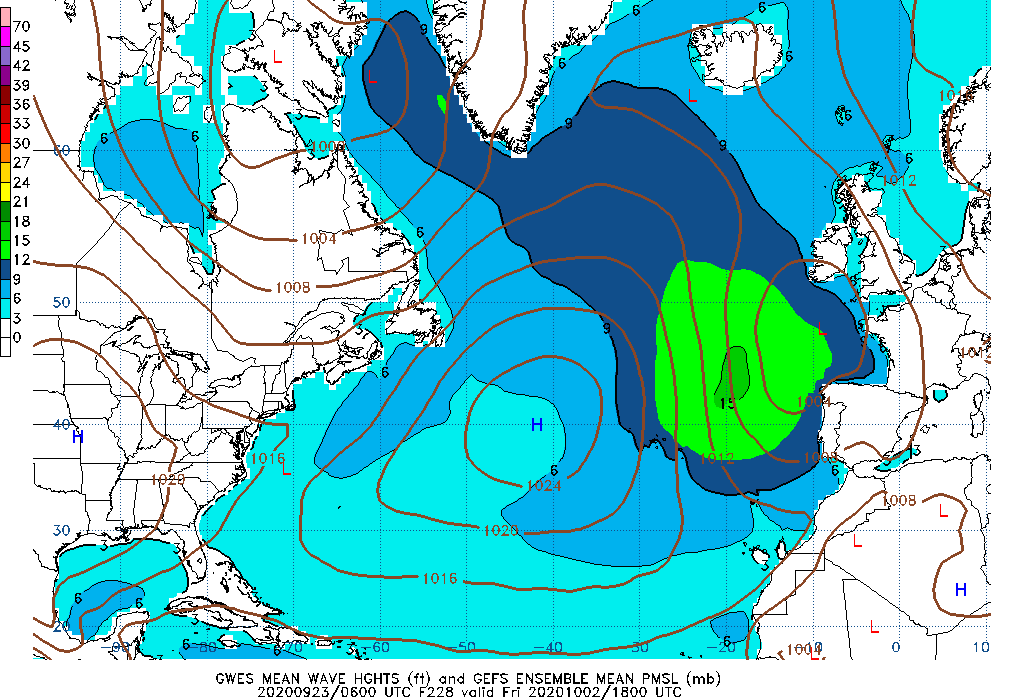228 Hour Wave Height mean 234 Hour Wave Height mean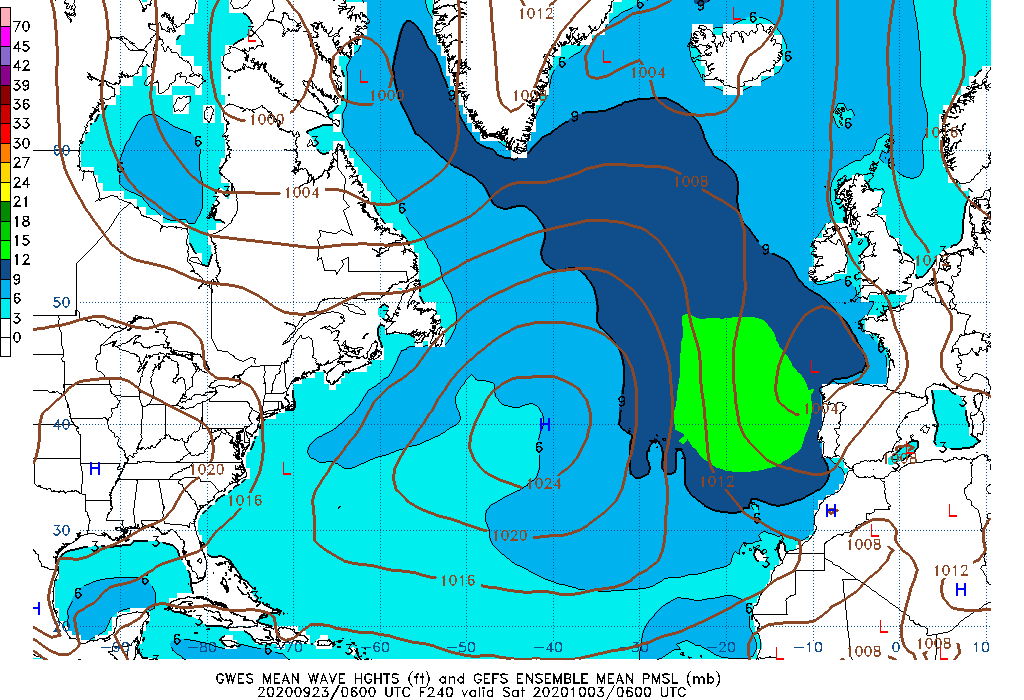240 Hour Wave Height mean

Notes on images above:

• The likelihood of exceedance is shown as a probability of 0 to 100 of waves heights (significant wave height) reaching or being greater than the chosen value.

• The 90th percentile represents the wave height value that 90% of the ensemble members are either less than or equal to (i.e., 18 feet).

• The 10th percentile is the wave height value that is represented by 10% of the contributing ensemble members.

• The mean is defined as the 50th percentile, that is, the wave height value represented reflects half the distribution is above and half is below this value.

• The maximum value is the highest value produced by any of the 21 ensemble members. It reflects the most severe conditions predicted by the ensemble prediction system.

The data are produced from the Global Ensemble Forecast System (GEFS) which is run every 6 hours and are created with 20 ensemble members.

Additional information for interpreting this data

More information is available from NOAA's Environmental Modeling Center (EMC).